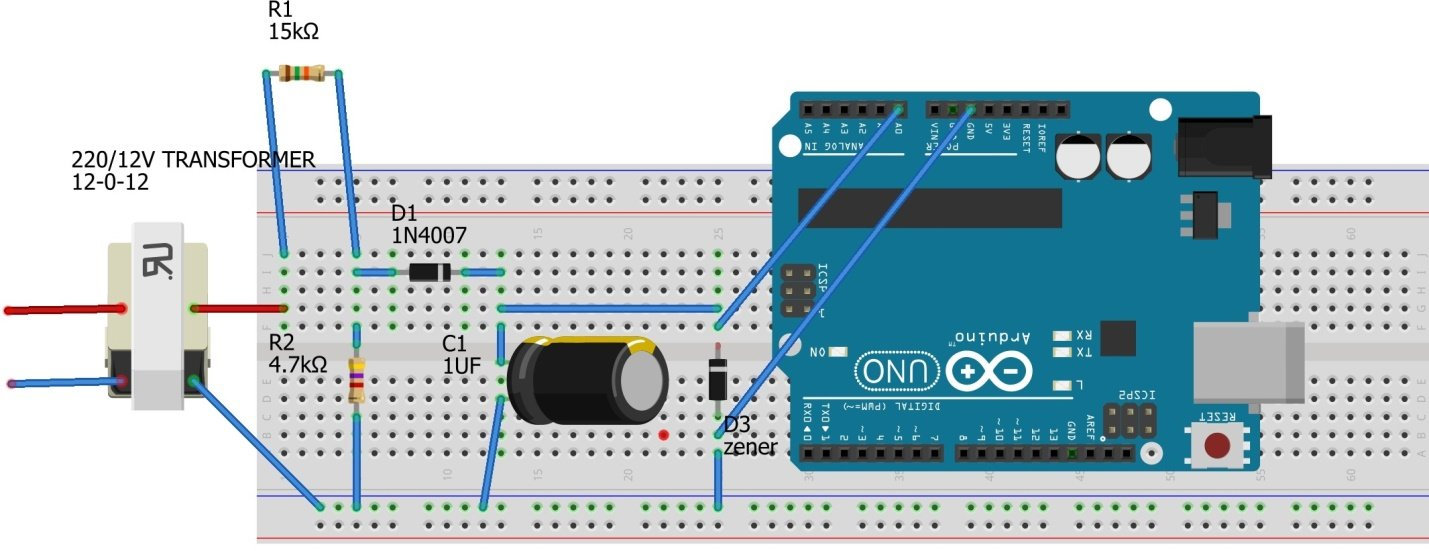# How To Make a Digital Voltmeter Using Arduino(Simplest)INTRODUCTION:

Voltmeters are required in almost every sector of electrical and electronics industry. And since Digital multimeters are easily available in the market, there is no big issue in buying one as it can measure all electrical quantities.

But the real problem arises when one wants to make his own analog voltmeter because making one is not easy. Whereas a digital voltmeter can be made easily using a microcontroller (We are going to use Arduino for this purpose). Arduino will take step-down ac voltage as input and displays the real ac voltage on the serial monitor. You can also use an LCD to display the voltage.

This circuit is capable of measuring a.c voltage as high as 311 volts (r.m.s). And also protects the microcontroller in case this a.c voltage increases beyond 311 volts.

If you prefer Video tutorials instead, then watch this video:

Watch this video to learn how to make this simplest digital voltmeter using arduino in 5 minutes .

COMPONENTS:

1.  One 12-0-12 transformer

2.  Resistors 10k; 4.7k.

3.  1N4007 diode

4.  1uf capacitor

5.   Zener diode(5v)

6. Arduino UNO

7. Connecting wires

CIRCUIT DIAGRAM:

CONNECTIONS:

High voltage side of transformer should be connected to direct ac supply, whereas low voltage side(side and middle wire)  is connected to the voltage divider circuit. The output from 4.7 k resistor is connected to the diode which rectifies the step-down ac voltage and gives it to the 1 uf capacitor which further removes any ripples present. This capacitor charges and provides an input voltage to the A0 pin of Arduino.

NOTE– 1.  As we know that Arduino cannot take negative voltage value as input, since the negative voltage can damage Arduino; input ac voltage must be rectified first. Hence diode is used to remove negative rail of voltage.  After that 1 u farad capacitor removes ripples present and thus provide the smooth voltage value to Arduino.

1.  Zener diode is used to protect Arduino from damaging if voltage across the capacitor exceeds 5v. When this happens Zener diode breakdowns and thus all current flows through Zener to ground thereby ensuring the safety of Arduino.

CODE:

 int x;// initializing variables float y;//   void setup() { pinMode(A0,INPUT); // pin A0 as input pin Serial.begin(9600);// begin serial communication between Arduino andlaptop }   void loop() { x=analogRead(A0);// assigning analog values from pin A0 to variable x y=(x* .304177);//  analog value(x) into corresponding  input ac supply value using this formula ( see working section)   Serial.print(”   analog input  ” ) ; // Serial.print(x) ; // print input analog value on serial monitor Serial.print(”   ac voltage  “) ; // Serial.print(y) ; // prints the ac value on Serial monitor Serial.println(); }

UNDERSTANDING CODE:

1. VARIABLE x:

X is the input analog value received (voltage) from pin A0 i.e.,

x =    pinMode (A0,INPUT) ;  // set pin a0 as input pin

2. VARIABLE y

Task of this variable is most important in whole program i.e., to convert the input analog value into the actual ac voltage from mains. As in the code:

y= (m*.304177) ; // convert into ac voltage

x or actual analog value is multiplied by .304177 because of the following reason:

This exact circuit is simulated in Proteus and the maximum voltage is found out by hit and trial method:

So by simulating the circuit, we observed that when input a.c voltage is 311 volts, 5 v or 1023 analog value is obtained at pin A0. This voltage is the maximum value that can be measured by this circuit. Above this voltage Zener diode will breakdown. Hence:

311-volt mains supply corresponds to 1023 analog value.

So any random analog value obtained is equal to (311/1023)*x ac volts, where x is obtained analog value.

Hence we arrive at this formula: y=(311/1023)*x volts or y=(x*.304177).

WORKING:

A) Transformer step-down the input ac voltage.

B) Voltage divider circuit ensures voltage less than 5 v across the 4.7k resistor and hence maximum input a.c volts as already seen in simulation must be 311 r.m.s volts.

C) Arduino receives this voltage as analog value from pin A0 and converts this value into the actual input a.c voltage by the formula: y=(x*.304177).

D) This value is printed on the serial monitor and thus we get the required value of input a.c voltage.

NOTE- to see this code in action, watch video at the beginning.

Printing required values on screen::

Two values are printed on screen or serial monitor of Arduino IDE as shown in the picture:

Serial.print(”   analog input  “) ; // this command specify name on top of the values to be printed which is “ analog input”

Serial.print(x);// this simply prints the input analog value

1. Actual value of ac voltage from mains as specified in the code:

Serial.print(”   ac voltage  “) ; // this command specify  name on top of the values to be printed which is “ ac voltage”

Serial.print(y) ;  // this simply prints the ac voltage value

### 2 Replies to “How To Make a Digital Voltmeter Using Arduino(Simplest)”

1.Jatin patel says:

Hi my name is jatin patel I like the method that you did,But I wanted to know that how much 12-0-12 ampr required for it?

1.Ankit Negi says:

Do you want to know the current rating of 12-0-12 transformer?
1 Amp is fine.